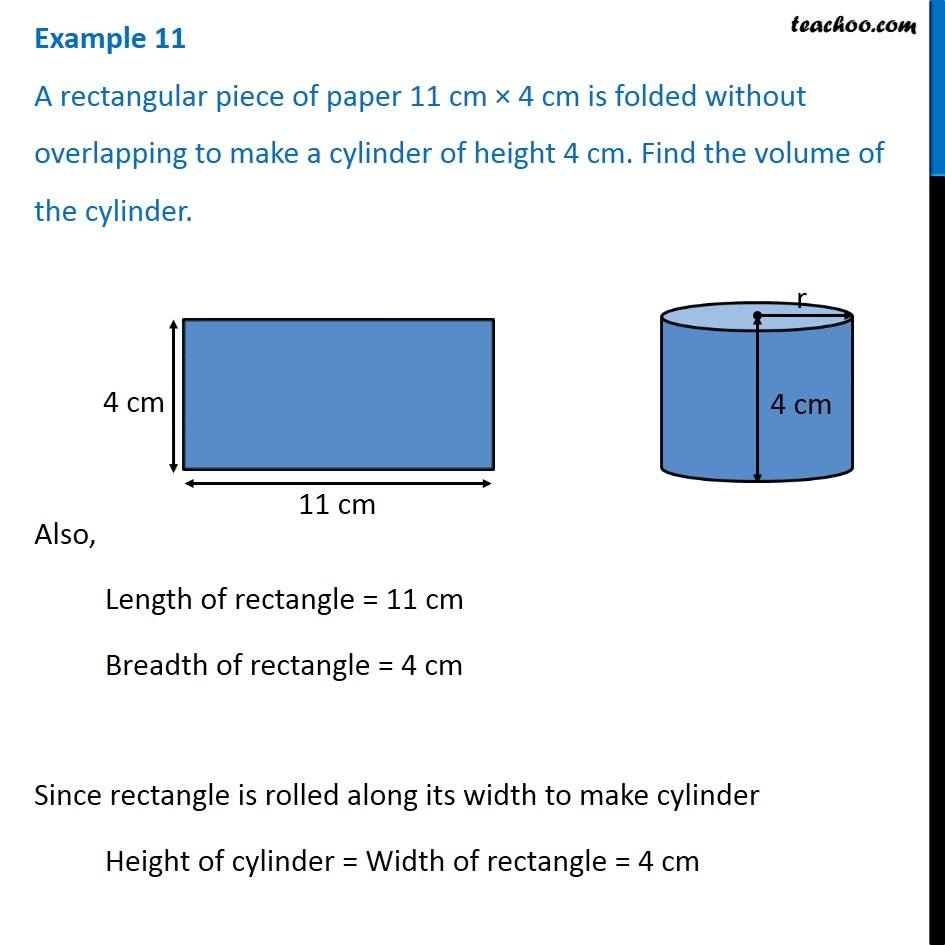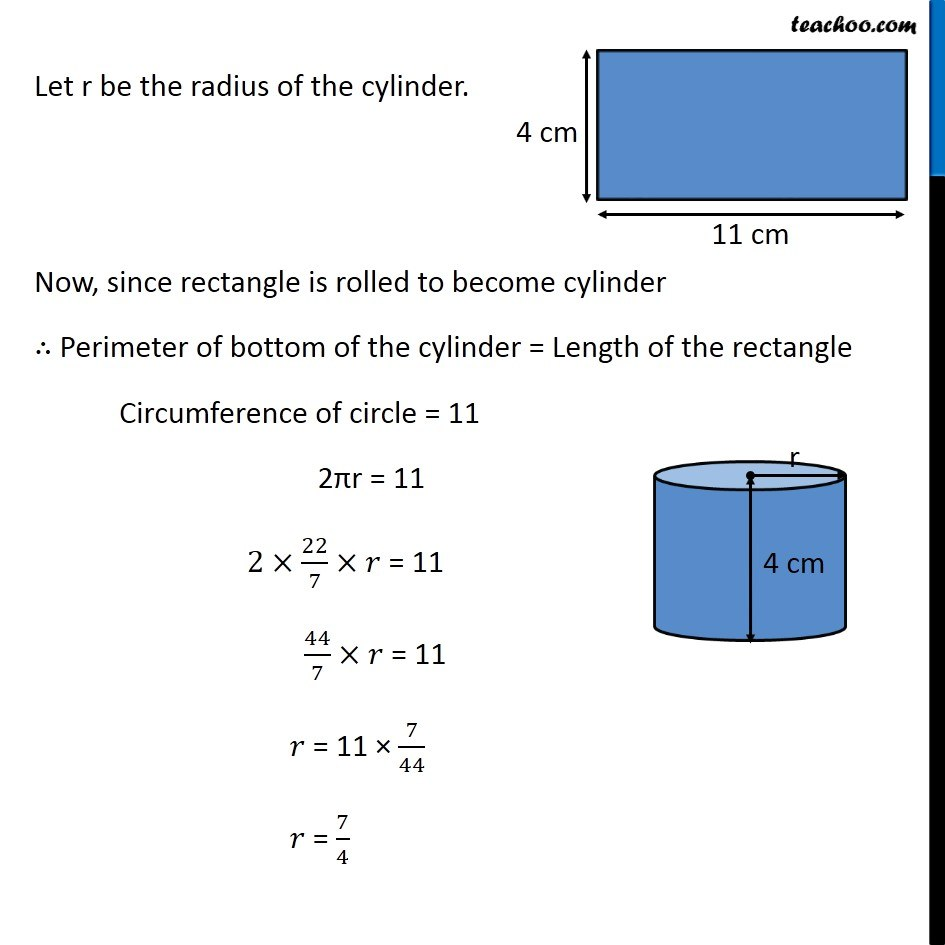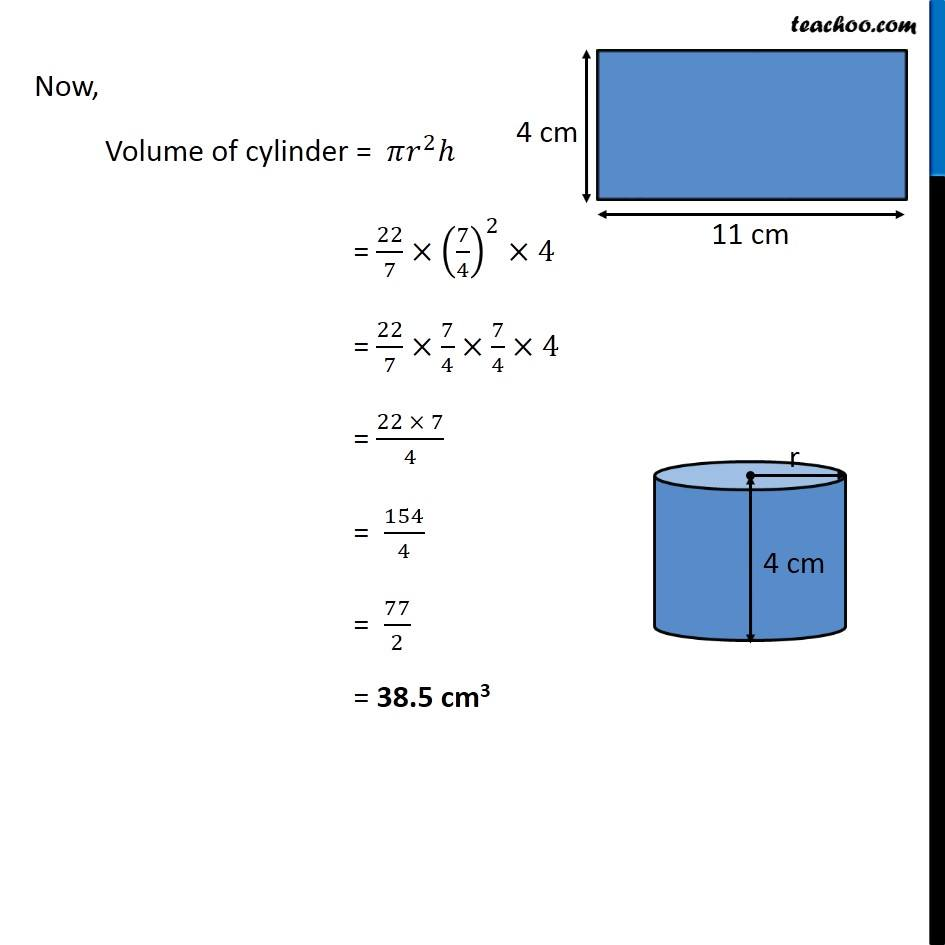Volume of Cylinder

Chapter 11 Class 8 Mensuration
Concept wiseIntroducing your new favourite teacher - Teachoo Black, at only ₹83 per month

### Transcript

Example 11 A rectangular piece of paper 11 cm × 4 cm is folded without overlapping to make a cylinder of height 4 cm. Find the volume of the cylinder. Also, Length of rectangle = 11 cm Breadth of rectangle = 4 cm Since rectangle is rolled along its width to make cylinder Height of cylinder = Width of rectangle = 4 cm Let r be the radius of the cylinder. Now, since rectangle is rolled to become cylinder ∴ Perimeter of bottom of the cylinder = Length of the rectangle Circumference of circle = 11 2πr = 11 2×22/7×𝑟 = 11 44/7×𝑟 = 11 𝑟 = 11 × 7/44 𝑟 = 7/4 Now, Volume of cylinder = 𝜋𝑟^2 ℎ = 22/7×(7/4)^2×4 = 22/7×7/4×7/4×4 = (22 × 7)/4 = 154/4 = 77/2 = 38.5 cm3